Next: Kinetic Gas Theory Up: Glow Discharges in Inert Previous: Glow Discharges in Inert   Contents   Index

## Gases and Ideal Gases

The gaseous state of matter is characterized by the fact that it fills always all the available space, means a gas has neither a specific shape nor a defined volume. Microscopical a gas consists out of individual particles with a specific volume and some distance between. The constituents can interact by elastic and inelasic collisions.

A gas is considered as ideal if it can be described just by the three macroscopic properties temperature, volume and pressure. The following relation must hold between the macroscopic variables of an ideal gas: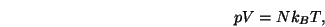(1.1)

whereis the Boltzmann constant,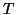the absolute temperature in Kelvin and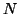denotes the total number of gas molecules/atoms. In materials processing usually the absolute number of particles is not as important as the particle density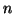:(1.2)

From the previous equation it is obvious that the gas density depends only on pressure and absolute temperature. By filling the above formula with numbers we get: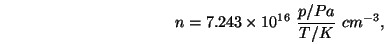(1.3)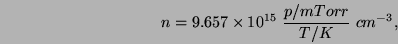(1.4)

where pressure and temperature are measured in Pascal and Kelvin, the second formula is the same just with the pressure given in mTorr, as this is still very commonly used, if you are active etching you what I am talking about, one equipment vendor likes Torr or mTorr the other Pascal and you are continously calculating how many mTorr is one Pascal, remember it is 7.5mTorr. To get more in touch with the numbers involved lets calculate the density at a typical processing pressure of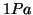and a temperature of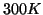. The resulting densityis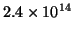particles per cubic centimeter. If we reduce the dimensions to the typical feature size in semiconductor manufacturing of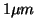then a cubic with this width contains just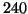particles.

The term ideal gas relates to the fact that the state does not depend on the kind of gas, all gases behave in the same way. In reality, especially at higher pressure and lower temperature, this is not always true, but under the typical conditions of material processing all gases can be treated as ideal. In the kinetic gas theory eqn.can be deduced from basic principles under the following boundary conditions:

• The total volume of the constituents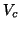is negligible compared to the volume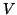in which the gas is enclosed,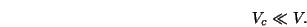(1.5)

This means also that most of the space is empty and the distance between the the particles is much bigger than their size.

• The particles interact just by elastic collisions, exchanging kinetic energy among them and with the wall.

From this it is quite clear why especially at low pressure most gases can be treated as ideal. As we saw in eqn.lower pressure means lower density. So less particles per volume lead to long distances and small interactions between them. As we will see in the next section on kinetic theory not the type or mass of the constituents is important, just the kinetic energy.

SubsectionsNext: Kinetic Gas Theory Up: Glow Discharges in Inert Previous: Glow Discharges in Inert   Contents   Index

back to www.gs68.de

Other important things: Legal Notice, Contact us

Copyright © 2001-2012 Spitzlsperger Technologies GmbH